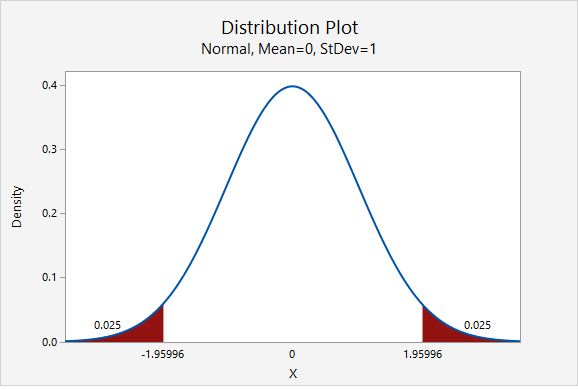# 8.1.1.1 - Normal Approximation Formulas

8.1.1.1 - Normal Approximation Formulas

For the following procedures, the assumption is that both $$np \geq 10$$ and $$n(1-p) \geq 10$$. When we're constructing confidence intervals $$p$$ is typically unknown, in which case we use $$\widehat{p}$$ as an estimate of $$p$$.

Note that $$n \widehat p$$ is the number of successes in the sample and $$n(1- \widehat p)$$ is the number of failures in the sample.

This means that our sample needs to have at least 10 "successes" and at least 10 "failures" in order to construct a confidence interval using the normal approximation method.

Below is the general form of a confidence interval.

General Form of Confidence Interval
$$sample\ statistic\pm\underbrace{(multiplier)\ (standard\ error)}_{\textbf{margin of error}}$$

The sample statistic here is the sample proportion, $$\widehat p$$. When using the normal approximation method the multiplier is taken from the standard normal distribution (i.e., z distribution).  And, the standard error is computed using $$\widehat p$$ as an estimate of $$p$$: $$\sqrt{\frac{\hat{p} (1-\hat{p})}{n}}$$. This leaves us with the following formula to construct a confidence interval for a population proportion:

Confidence Interval of $$p$$: Normal Approximation Method
$$\underbrace{\widehat{p}}_{\text{sample statistic}} \pm \overbrace{z^{*}}^{\text{multiplier}} \underbrace{\left (\sqrt{\frac{\hat{p}(1-\hat{p})}{n}}\right)}_{\text{standard error}}$$

## Finding the z* Multiplier

The value of the $$z^*$$ multiplier depends on the level of confidence. The multiplier for the confidence interval for a population proportion can be found using the standard normal distribution [i.e., z distribution, N(0,1)]. The most commonly used level of confidence is 95%. As shown on the probability distribution plot below, the multiplier associated with a 95% confidence interval is 1.960, often rounded to 2 (recall the Empirical Rule and 95% Rule).Below is a table of frequently used $$z^*$$ multipliers.

Confidence level and corresponding multiplier.
Confidence Level $$z^*$$ Multiplier
90% 1.645
95% 1.960, often rounded to 2
98% 2.327
99% 2.576

The value of the multiplier increases as the confidence level increases. This leads to wider intervals for higher confidence levels. We are more confident of catching the population value when we use a wider interval.

# 8.1.1.1.1 - Video Example: PA Residency

8.1.1.1.1 - Video Example: PA Residency

# 8.1.1.1.2 - Video Example: Dog Ownership

8.1.1.1.2 - Video Example: Dog Ownership

In Spring 2016, a sample of 522 World Campus students were surveyed and asked if they own a dog. Of the 522 students in the sample, 273 said that they did have a dog. Construct a 95% confidence interval for the proportion of all World Campus students who have a dog.

# 8.1.1.1.3 - Video Example: Books

8.1.1.1.3 - Video Example: Books

# 8.1.1.1.4 - Example: Retirement

8.1.1.1.4 - Example: Retirement

In a representative sample of 1168 American adults, 747 said they were not financially prepared for retirement. Let's construct a 95% confidence interval to estimate the proportion of all American adults who are not financially prepared for retirement.

First, we need to check our assumptions that both $$n\widehat p \geq 10$$ and $$n(1-\widehat p) \geq 10$$.

$$\widehat{p}=\frac{747}{1168}=0.640$$

$$np=1168 (0.640) = 747$$ and $$n(1-p)=1168(1-0.640)=421$$

Both are greater than 10, so this assumption has been met. This means we can use the normal approximation method to construct this confidence interval.

Next, we can compute the standard error.

$$SE=\sqrt{\frac{\hat{p} (1-\hat{p})}{n}}=\sqrt{\frac{0.640 (1-0.640)}{1168}}=0.014$$

The $$z^*$$ multiplier for a 95% confidence interval is 1.960

The formula for a confidence interval for a proportion is $$\widehat{p}\pm z^* (SE)$$

$$0.640\pm 1.960(0.014)=0.640\pm0.028=[0.612, \;0.668]$$

We are 95% confident that between 61.2% and 66.8% of all American adults are not financially prepared for retirement.

What if we wanted a 99% confidence interval?

Let’s think about how our interval will change. The 99% confidence interval will be wider than the 95% confidence interval. In order to increase our level of confidence, we will need to expand the interval.

In terms of computing the 99% confidence interval, we will use the same point estimate $$\widehat{p}$$ and the same standard error. Only the multiplier will change. From the plot below, we see that the $$z^*$$ multiplier for a 99% confidence interval is 2.576.$$99\%\;C.I.:\;0.640\pm 2.576 (0.014)=0.0640\pm 0.036=[0.604, \; 0.676]$$

We are 99% confidence that between 60.4% and 67.6% of all American adults are not financially prepared for retirement.

  Link ↥ Has Tooltip/Popover Toggleable Visibility